## InversionInversion is the process of transforming points to their Inverse Points. This sort of inversion was first systematically investigated by Jakob Steiner. Two points are said to be inverses with respect to an Inversion Circle with Inversion Center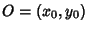and Inversion Radiusifandare line segments symmetric aboutand tangent to the Circle, andis the intersection ofand. The curve to which a given curve is transformed under inversion is called its Inverse Curve.

Note that a point on the Circumference of the Inversion Circle is its own inverse point. The inverse points obey(1)

or(2)

whereis called the Power. The equation for the inverse of the pointrelative to the Inversion Circle with Inversion Center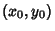and inversion radiusis therefore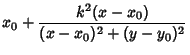(3)(4)

In vector form,(5)

Any Angle inverts to an opposite Angle.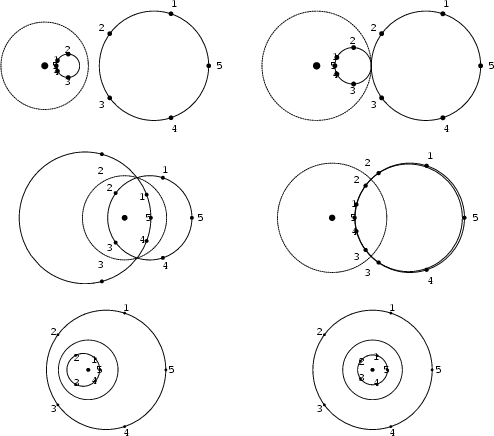Treating Lines as Circles of Infinite Radius, all Circles invert to Circles. Furthermore, any two nonintersecting circles can be inverted into concentric circles by taking the Inversion Center at one of the two limiting points (Coxeter 1969), and Orthogonal Circles invert to Orthogonal Circles (Coxeter 1969).

The inverse of a Circle of Radiuswith Centerwith respect to an inversion circle with Inversion Center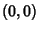and Inversion Radiusis another Circle with Centerand Radius, where(6)The above plot shows a checkerboard centered at (0, 0) and its inverse about a small circle also centered at (0, 0) (Dixon 1991).

See also Arbelos, Hexlet, Inverse Curve, Inversion Circle, Inversion Operation, Inversion Radius, Inversive Distance, Inversive Geometry, Midcircle, Pappus Chain, Peaucellier Inversor, Polar, Pole (Geometry), Power (Circle), Radical Line, Steiner Chain, Steiner's Porism

References

Courant, R. and Robbins, H. Geometrical Transformations. Inversion.'' §3.4 in What is Mathematics?: An Elementary Approach to Ideas and Methods, 2nd ed. Oxford, England: Oxford University Press, pp. 140-146, 1996.

Coxeter, H. S. M. Inversion in a Circle'' and Inversion of Lines and Circles.'' §6.1 and 6.3 in Introduction to Geometry, 2nd ed. New York: Wiley, p. 77-83, 1969.

Coxeter, H. S. M. and Greitzer, S. L. Geometry Revisited. Washington, DC: Math. Assoc. Amer., pp. 108-114, 1967.

Dixon, R. Inverse Points and Mid-Circles.'' §1.6 in Mathographics. New York: Dover, pp. 62-73, 1991.

Johnson, R. A. Modern Geometry: An Elementary Treatise on the Geometry of the Triangle and the Circle. Boston, MA: Houghton Mifflin, pp. 43-57, 1929.

Lockwood, E. H. Inversion.'' Ch. 23 in A Book of Curves. Cambridge, England: Cambridge University Press, pp. 176-181, 1967.

Ogilvy, C. S. Excursions in Geometry. New York: Dover, pp. 25-31, 1990.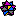Weisstein, E. W. Plane Geometry.'' Mathematica notebook PlaneGeometry.m.